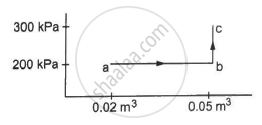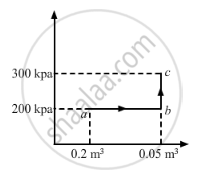Advertisement Remove all ads

# A Substance is Taken Through the Process Abc as Shown in Figure. If the Internal Energy of the Substance Increases by 5000 J and a Heat of 2625 Cal is Given to the System, Calculate the Value of J. - Physics

Sum

A substance is taken through the process abc as shown in figure. If the internal energy of the substance increases by 5000 J and a heat of 2625 cal is given to the system, calculate the value of J.Advertisement Remove all ads

#### Solution

Given:-

Heat given to the system, ∆Q = 2625 cal

Increase in the internal energy of the system, ∆U = 5000 JFrom the graph, we get

W = Area of the rectangle formed under line ab + Area under line bc

For line BC:-

Change in volume = 0

WBC = P Δ V = 0

∆W = Area of the rectangle

∆W = 200 × 103 × 0.03

= 6000 J

We know,

∆Q = ∆W + ∆U

⇒ 2625 cal = 6000 J + 5000 J

$\Rightarrow J = \frac{11000}{2625} = 4 . 19 \text{ J/cal}$

Concept: Heat, Internal Energy and Work
Is there an error in this question or solution?
Advertisement Remove all ads

#### APPEARS IN

HC Verma Class 11, Class 12 Concepts of Physics Vol. 2
Chapter 4 Laws of Thermodynamics
Q 12 | Page 63
Advertisement Remove all ads
Advertisement Remove all ads
Share
Notifications

View all notifications

Forgot password?